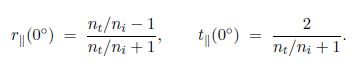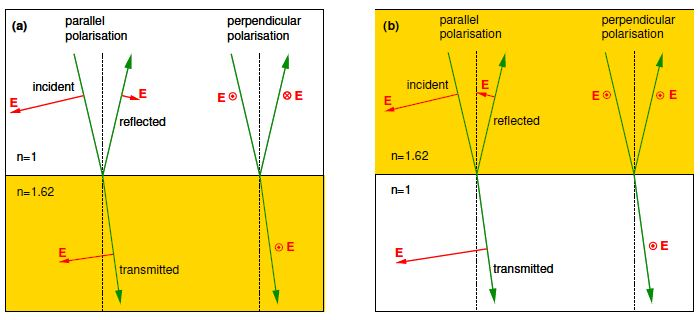# Explain Normal Incidence in Amplitude Coefficients

For normal incidence the plane of incidence is undefined and the results we have obtained for the cases of parallel and perpendicular polarisations must therefore become identical as θi → 0. For perpendicular polarisation the amplitude coefficients for normal incidence arewhereas for parallel polarisation Equations becomeFrom these Equations (and θi = 0°) we see that r (0°) = — r (0°), but for normal incidence perpendicular and parallel polarisations should be identical!

So what is going on? To understand, we must remember that these coefficients and phase shifts are defined relative to the electric field directions of the incident, reflected and transmitted waves. When these amplitude reflection coefficients coefficients are applied to the electric fields of the reflected and transmitted waves for a small angle of incidence the fields are as in below Figure.Figure: Electric field directions for near-normal incidence for (a) external reflection off flint glass and (b) internal reflection within flint glass.

For external reflection (Fig. a) at normal incidence the electric field direction of the reflected wave is opposite to that of the incident wave, whereas for internal reflection (Fig. b) the electric field direction is in the same direction as that of the incident wave. Hence, for reflection at normal incidence there is a phase shift of 180° on reflection for external reflection, but no phase shift for internal reflection irrespective of polarisation.